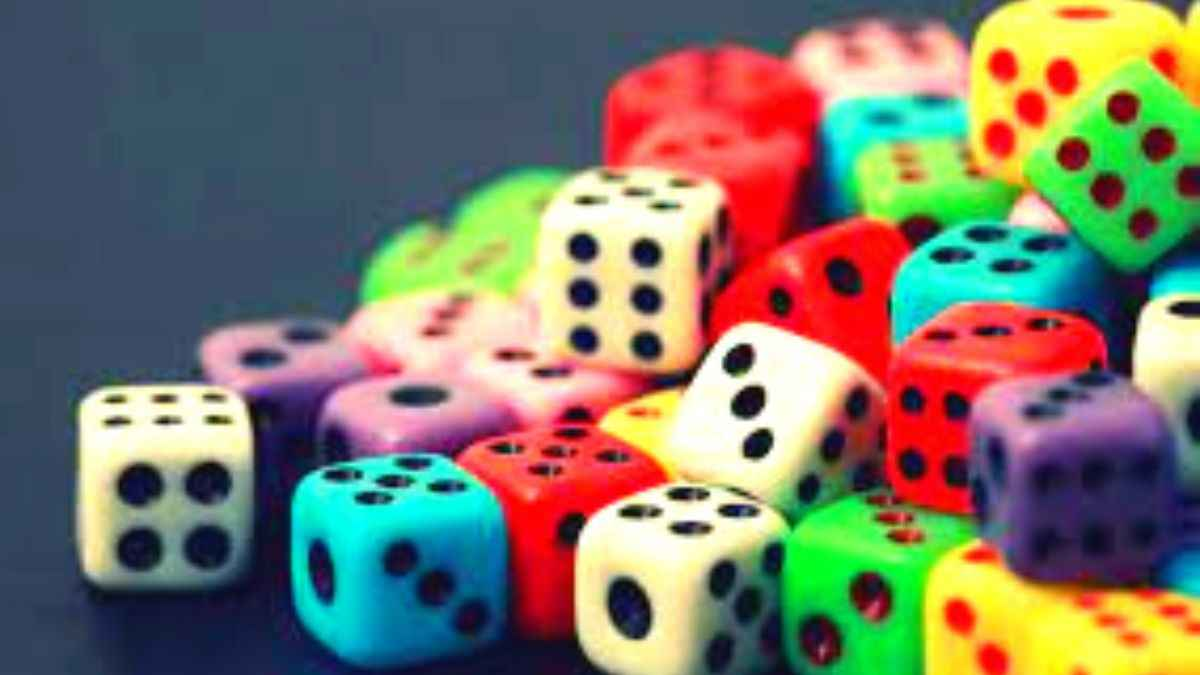# Attempting to answer these math riddles on dice is like rolling your dice!

Here are some exciting math riddles on dice. Try solving them if you can.Have you ever played with dice? Well, of course, you have! You spent a great deal of your time in your childhood playing dice. You must have played “Shut the box” in your childhood. The “Liar’s Dice” is also a fun game. And oh, how can you forget the famous “Bunco”?

Try these fun math riddles based on dice!

## Math Riddle 1:

Two coins are tossed, finding the probability that two heads are obtained. Note: Each coin has two possible outcomes H (heads) and T (Tails).

## Math Riddle 2:

A die is rolled and a coin is tossed, find the probability that the die shows an odd number and the coin shows a head.

## Math Riddle 3:

A die is rolled, find the probability that an even number is obtained.

Where have you kept your favorite dice?

### Math Riddle 1:

Two coins are tossed, finding the probability that two heads are obtained. Note: Each coin has two possible outcomes H (heads) and T (Tails).

The sample space S is given by.

S = {(H,T),(H,H),(T,H),(T,T)}

Let E be the event "two heads are obtained".

E = {(H,H)}

We use the formula of classical probability.

P(E) = n(E) / n(S) = 1 / 4

### Math Riddle 2:

A die is rolled and a coin is tossed, find the probability that the die shows an odd number and the coin shows a head.

Let H be the head and T be the tail of the coin. The sample space S of the experiment described in question 5 is as follows

S = { (1,H),(2,H),(3,H),(4,H),(5,H),(6,H)

(1,T),(2,T),(3,T),(4,T),(5,T),(6,T)}

Let E be the event "the die shows an odd number and the coin shows a head". Event E may be described as follows

E={(1,H),(3,H),(5,H)}

The probability P(E) is given by

P(E) = n(E) / n(S) = 3 / 12 = 1 / 4

### Math Riddle 3:

A die is rolled, find the probability that an even number is obtained.

Let us first write the sample space S of the experiment.

S = {1,2,3,4,5,6}

Let E be the event "an even number is obtained" and write it down.

E = {2,4,6}

We now use the formula of classical probability.

P(E) = n(E) / n(S) = 3 / 6 = 1 / 2

Get the latest General Knowledge and Current Affairs from all over India and world for all competitive exams.
खेलें हर किस्म के रोमांच से भरपूर गेम्स सिर्फ़ जागरण प्ले पर